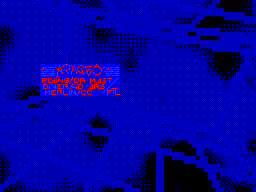Scenergy #02 31 декабря 1999Coding - 16-битная процедура быстрого возведения в степень.FAST 16-bit SQUARE ROOT

This  is  nice,  but what about arbitrary numbers which are not
powers of two? Fortunatelly, there is nice trick which allows us
to look at arbitrary number this way:

X = 2^N * M

Where:
N is number of bits needed to represent number X
M is mantissa of X and lies in interval <0, 1>

Thinking this way, we can say:

128 = 2^8 * 0.5
40 = 2^6 * 0.625

Since  we  are  experienced  in  mathematics  [are we?], we can
calculate sqrt(X) easily:

sqrt(X) = 2^(N/2) * sqrt(M)

For example:

sqrt(144) = sqrt(2^8 * 0.5625) = 2^4 * sqrt(0.5625) =
= 2^4 * 0.75 = 12

Voila!  It  works!  Let's  summarize  it  and write square root
algorithm:

1) Find out N [number of bits we need to represent number X].
2) Calculate mantissa M.
3) Take sqrt(M) from table.
4) Take N/2 highest bits from mantissa to get result.

One may ask how do we calculate the mantissa. Answer is simple.
We just take highest 8 bits of number X [highest 8 bits does not
mean  higher byte!]. Then we make one lookup to SQRT table which
is built this way:

for i = 0 to 255
SQRT[i] = 256 * sqrt(i / 256)

Few  more  questions  appear.  Is 8 bits enough? Is square root
stored  with  enough  precision?  Yes.  Since we are calculating
square  root  of  16-bit number, result will be surely 8-bit. In
addition,  sqrt(M)  is even bigger number than M [but still lies
in  interval <0, 1>]. Smart reader can also ask: "What if N will
be  odd?  How  we  half it then?". Again, solution is simple. We
will take nearest higher value as N. For example:

24 = 2^5 * 0.75 => 2^6 * 0.375

That's  all  about  basis  of  this  method.  Now  we can start
developing routine. The main trick is to put highest 8 bits of X
into  one byte to take mantissa [as these bits can lie partly in
higher  byte  and  partly  in  lower  byte  of X]. I solved this
problem  by  set  of tables. First table shifts higher byte of X
into leftmost position. Few examples should make it clear:

01 -> 10 ... shifted left 4 times
^
00110101 -> 11010100 ... shifted left 2 times
^^
0110 -> 110 ... shifted left 5 times
^
Now  we  need just to add few highest bits from lower byte of X
at  highlighted positions. This is done by set of masking tables
[there  are eight of them; each will return 0-7 highest bits for
given number]. Let me show some examples:

Table 3

1 -> 0111 ... three highest bits
10100110 -> 0101
^^^
Table 5

1 -> 0001 ... five highest bits
10011010 -> 00010011
^

This  table  set can be also used to get final N/2 highest bits
from mantissa. Of course, we must somehow remember how many bits
we  shifted left, because we must know how many bits to add from
lower byte. This is where next table come from. For each shifted
number  it  contains  pointer to related masking table. I am not
going  to  confuse  you  with  more explanations, so look at the
final code:

BC - number to proccess

ld      h,SHIFT       ; we are going to shift higher byte of X
ld      l,b
ld      a,(hl)        ; shift it!
inc     h
ld      b,(hl)        ; get pointer to N/2 masking table
inc     h
ld      h,(hl)        ; get pointer to masking table
ld      l,c
or      (hl)          ; add lower bits [calculate the mantissa]
ld      h,SQRT
ld      l,a
ld      c,(hl)        ; get square root of mantissa
ld      a,(bc)        ; get N/2 highest bits from mantissa

Summa  summarum,  76T and just 3K of tables. Would you ever say
that  this  linear  piece  of  code  can perform square root? Of
course,  some  extremists [those like me] can save another 3T by
changing  "ld h,SQRT" to "inc h" and having SQRT table stored in
memory  several  times. It is upon you to decide if 3T are worth
of additional 2K. Oh! I forgot table generator, so you will have
to  make  it  by  yourself.  It will help you to understand this
method  better  and  maybe  you  will  find  something even more
usable. Happy coding!

-baze-3SC->

Другие статьи номера:

Warez Pack - описание импортной демки 'no work'.

Похожие статьи:

В этот день...   27 января